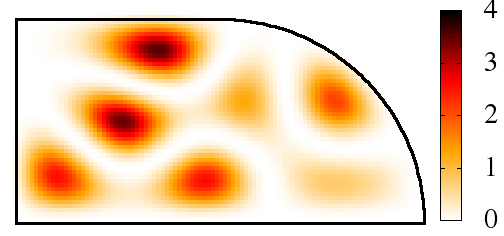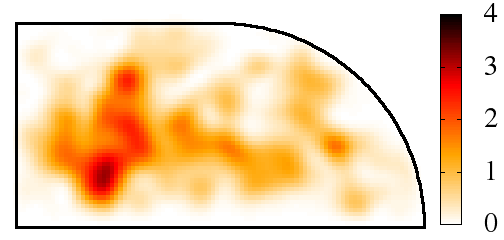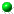Dynamical thermalization
of Bose-Einstein condensate
in Bunimovich stadium
by L.Ermann, E.Vergini and D.L.Shepelyansky
arXiv:1505.05130[cond-mat.quant-gas]Time evolution of initial linear eigenstate m=10 for the Gross-Pitaevskii equation at nonlinearity beta=10 (left t=0, right t=40; color bar shows amplitude of wavefunction)

 ` `Article download arXiv-v1 (May 2015): (here); EPL published (here)All figs in gzip format (here )Video of time evolution from t=0 to t=40 of initial state m=5, beta=10 (color shows absolute value of wavefunction; use Firefox) (video)Video of time evolution from t=0 to t=40 of initial state m=10, beta=10 (color shows absolute value of wavefunction; use Firefox) (video)Related web site for the GPE in Sinai oscillator (here) This webpage is created at May 15, 2015 and is maintained by L.Ermann and D.L.Shepelyansky